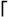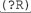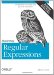# Section 10.5. Recursive Expressions

### 10.5. Recursive Expressions

Most aspects of the preg engine's flavor are covered as general topics in Chapter 3, but the flavor does offer something new in its interesting way of matching nested constructs: recursive expressions.

The sequence(?R)means "recursively apply the entire expression at this point," while(? num )sequence means "recursively apply the sequence within the numbered set of capturing parentheses at this point." The named-capture version of the latter uses a(?P> name )notation.

The next few sections show some common uses for recursion. Recursion also plays a central role in the extended "tagged data" example, which starts on page 481.

#### 10.5.1. Matching Text with Nested Parentheses

The quintessential recursive example is to match text containing nested sets of parentheses. Here's one way:(?: [^()]++ \(\) )*This expression matches any number of two alternatives. The first alternative,[^()]++, matches everything except parentheses. This alternative requires its possessive version of + to avoid a "neverending match (˜ 226), due to the outer(?:‹)*enclosing it.

The other alternative,\((?R)\), is where things get interesting. The second alternative matches a pair of parentheses, with anything (as long as any parentheses are properly nested) in between. The "anything in between part is exactly what the overall regex is trying to match, which is why we can simply use(?R)to apply the current overall regex, recursively.

This expression works fine on its own, but be very careful adding anything to it, because anything added is also applied recursively during calls to(?R).

For example, consider using this expression to validate that an entire string has no unbalanced parentheses. You might be tempted to wrap it in^‹\$to enforce the notion of "the whole string." That would be a mistake, since the added line anchors would certainly cause the recursive calls, applied in the middle of the string, to fail.

##### 10.5.1.1. Recursive reference to a set of capturing parentheses

The(?R)construct makes a recursive reference to the entire regular expression, but a reference to a subset of the expression is possible with the(? num )construct. It makes a recursive reference to the subexpression contained within the numth set of capturing parentheses. [] Taking(? num )to its logical start,(?0)is a synonym for(?R).

[] Strictly speaking,(? num )need not be a recursive reference, that is, the(? num )construct itself need not necessarily be part of the subexpression contained within the num th set of capturing parentheses. In such cases, the reference can be considered more of a "subroutine call."

We can use a limited reference like this to solve the problem posed in the previous section: before adding^‹\$, we wrap the main part of the regex in capturing parentheses, then use(?1)where(?R)currently exists. The capturing parentheses are added to mark the subexpression that(?1)refers to, which, you might recall, is exactly the expression we had in the previous section, which matched nested parentheses. The^‹\$are added outside those parentheses, which is how we avoid applying them recursively:^( )\$The underlined part of the regex is within the first set of capturing parentheses, so that's what is reapplied each time(?1)is reached.

Here's our regex in a sample PHP snippet that reports whether the text within the \$text variable is balanced or unbalanced:

` if (preg_match('/^ ( (?: [^()]++ \(  (?1)  \) )*) \$/x', \$text))         echo "text is balanced\n";     else         echo "text is unbalanced\n"; `

##### 10.5.1.2. Recursive reference via named capture

If the subexpression to be called recursively has been wrapped with named parentheses (˜ 138), you have the option to use the (?P> name ) notation for the recursive reference, rather than the (? num ) notation we've seen so far. With this notation, our example might become:

`^  (?P<stuff>  (?: [^()]++\  ((?P>stuff)  \))*  )  \$. `

That expression may look complicated, but we can easily make it more readable with the x pattern modifier (˜ 446):

` \$pattern = '{     #  The regular expression begins here  ...     ^        (?P<stuff>             #  Everything within this set of parentheses is named "stuff."  (?:             [^()]++                   #  anything not parentheses  \( (?P>stuff) \)       #  an open paren, more "stuff," and finally a  close paren  .         )*     )     \$     #  This is the end of the regular expression  .     }x'; #  The 'x' here is a preg pattern modifier  .     if (preg_match(\$pattern, \$text))         echo "text is balanced\n";     else         echo "text is unbalanced\n"; `

##### 10.5.1.3. More on possessive quantifiers

I'll make one final comment on the use of possessive quantifiers in the original expression. If the outer(?:‹) *were possessive, the inner[^()]++need not be. In order for this expression to stay out of the neverending-match pit, one or the other (or both) must be possessive. If possessive quantifiers and atomic parentheses (˜ 259) were not available, youd have to remove the quantifier from the first alternative altogether:(?: [^()] <\(\) )*This would be less efficient, but at least it wouldn't be a neverending match. To regain efficiency, you could apply the "unrolling the loop" technique covered in Chapter 6 (˜ 261), which results in[^()]* (?: \((?R)\) [^()]* )*#### 10.5.2. No Backtracking Into Recursion

An important aspect of the preg flavor's recursion semantics is that it treats everything matched by recursion as if it were matched within atomic parentheses (˜ 259). That means that if recursion matches something that must ultimately be partially "unmatched" to achieve overall success, it won't happen (resulting in an overall failure).

The "partially" in the middle of that last sentence is important, because the entire text matched via a recursive call can, as a whole unit, be unmatched via backtracking. What recursion disallows is backtracking to a point back within the recursive call.

#### 10.5.3. Matching a Set of Nested Parentheses

We've seen how to match a line that has no unbalanced parentheses, so, for completeness, I'd like to show how to explicitly match a balanced set of parentheses (possibly containing additional nested sets within):\( (?: [^()]++ <(?R) )* \)This example uses the same ingredients as the previous one, but it is arranged a bit differently. As before, if you wish to use this as part of a larger expression, you need to wrap it in capturing parentheses and change(?R)to a recursive reference to a particular subexpression, such as(?1)(using the number appropriate to where the added capturing parentheses fall in the overall regex).Mastering Regular Expressions
ISBN: 0596528124
EAN: 2147483647
Year: 2004
Pages: 113

Similar book on Amazon